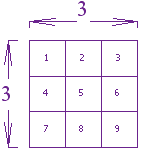# Square and square roots

## SQUARE NUMBERS

Roots" (or "radicals") are the "opposite" operation of applying exponents; you can "undo" a power with a radical, and a radical can "undo" a power. For instance, if you square 2, you get 4, and if you "take the square root of 4", you get 2; if you square 3, you get 9, and if you "take the square root of 9", you get 3:The "" symbol is called the "radical"symbol. The expression "√9 " is read as "root nine", "radical nine", or "the square root of nine".

If a natural number m can be expressed as n2, where n is also a natural number, then m is square number.

e.g., 22 = 2 × 2 = 4

9 = 3 × 3 = 32 etc.

### PERFECT SQUARES:

The numbers 1, 4, 9, 16,..... are square numbers. These numbers are also called perfect squares.

A perfect square numbers end with 0, 1, 4, 5, 6 or 9 at unit's place.

• If a number end with 2, 3, 7 or 8 at unit's place, it can never be a perfect square.
• If a number has 1 or 9 in the unit's place, then it's square ends in 1.
• When a square number ends in 6, the number whose square it is will have either 4 or 6 in unit's place.
• Squares of even numbers are always even.
• Squares of odd numbers are always odd.e.g.

question What is 3 squared?

 3 Squared == 3 × 3 = 9

"Squared" is often written as a little 2 like this:This says "4 Squared equals 16"(the little 2 says the number appears twice in multiplying)

### SQUARES FROM 12 TO 62:

 1 Squared = 12 = 1 × 1 = 1 2 Squared = 22 = 2 × 2 = 4 3 Squared = 32 = 3 × 3 = 9 4 Squared = 42 = 4 × 4 = 16 5 Squared = 52 = 5 × 5 = 25 6 Squared = 62 = 6 × 6 = 36

### NEGATIVE NUMBERS:

You can also square negative numbers.

question 1. What happens when you square (-5) ?

Sol. (-5) × (-5) = 25 (because a negative times a negative gives a positive)

When you square a negative number you get a positive result.

Just the same as if you had squared a positive number:

(For more detail read Squares and Square Roots in Algebra)

Note: if someone says "minus 5 squared" do you:

### You get different answers:

Square 5, then do the minus: Square (-5):

-(5×5) = -25, (-5)×(-5) = +25

Always make it clear what you mean, and that is what the "( )" are for. NCERT solutions for class 8 Maths  prepared by Physics Wallah will help you to solve your NCERT text book exercise.